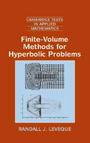# Finite Volume Methods for Hyperbolic Problems

## by Randall J. LeVeque.Introduction
This is an excellent introduction into finite volume methods for solving conservation laws. The book is divided into three main parts: Part I deals with linear equations in predominately one spatial dimension, Part II introduces nonlinear equations again in one spatial dimension, while Part III introduces multidimensional problems. Beginning with an introduction to the mathematics of these partial differential equations, including all the basic hyperbolic theory for linear equations (characteristics, Riemann problems, definition, and examples), LeVeque then presents the basics of finite volume methods for the integration of conservation laws. Extensions to these basics in terms of high resolution methods such as the use of limiters in multidimensional up-winding is then presented. The nonlinear portion of the book begins with the mathematics of nonlinear scalar conservation laws, the application of finite volume methods for their numerical solution, extensions to systems of equations, the nonlinear Riemann problem, non-classical hyperbolic systems, and finally concludes with a chapter on equations with source terms. The third part of the book deals with multidimensional hyperbolic problems and numerical methods both in scalar and vector form.

The book is exceptionally well written and the problems are worth subsequent study. If you work in the area of hyperbolic systems you must read this book.

One of the great bonuses that this book contains is that it includes a description and introduction on the use of the CLAWPACK software. The CLAWPACK of codes is a large collection of FORTRAN 77 codes for solving a large number of hyperbolic systems (both conservative and nonconservative forms). Included is a "getting started" section which can help new users become familiar with the codes and get them up and solving problems very quickly.

Solution & Notes:
I am in the process of transcribing my notes from this book into a solutions manual. You can find my first attempts at this process below. As always, please report any errors that may remain.
• My most recently typeset notes and problem solutions can be found here:
Code for problems from the book:
• Chapter 3 (Characteristics and Riemann Problmes for Linear Hyperbolic Equations):
• prob_3_1.m (phase plane solutions to linear Riemann problems)
• riemann2x2.m (solves a 2x2 linear Riemann problem)
• prob_3_3_b.m (plots the x-t solution for this linear Riemann problem)
• prob_3_4.m (plots the region in x-t plane where solution for this linear Riemann change)
• Chapter 5 (Introduction to the CLAWPACK software):
• Problem 5.1 (visualization of the solution to a 1-d advection flow problem with the upwind method)
• Problem 5.2 (the upwind method with Courant number larger than 1)
• Problem 5.3 (a pure left going pressure pulse)
• Chapter 9 (Variable-Coefficient Linear Equations):
• Chapter 13 (Nonlinear Systems of Conservation Laws):
• The Shallow Water Equations:
• prob_13_2.m (rarefaction wave curves for Example 13.5 plotted in the h-u plane)
• prob_13_4.m (phase plane solution of a one-shock/two-shock collision)
• The p-System:
• prob_13_11_b.m (plots the Hugoniot/Integral curves for Problem 13.11 Part b)
• prob_13_11_c.m (plots the phase plane solution for an example Riemann problem)
• prob_13_11_d.m (plots the phase plane solution for Riemann problem with no solution)
• prob_13_12.m (plots the solution for the Riemann problem in the x-t plane)
• Chapter 16 (Some Nonclassical Hyperbolic Problems):

John Weatherwax